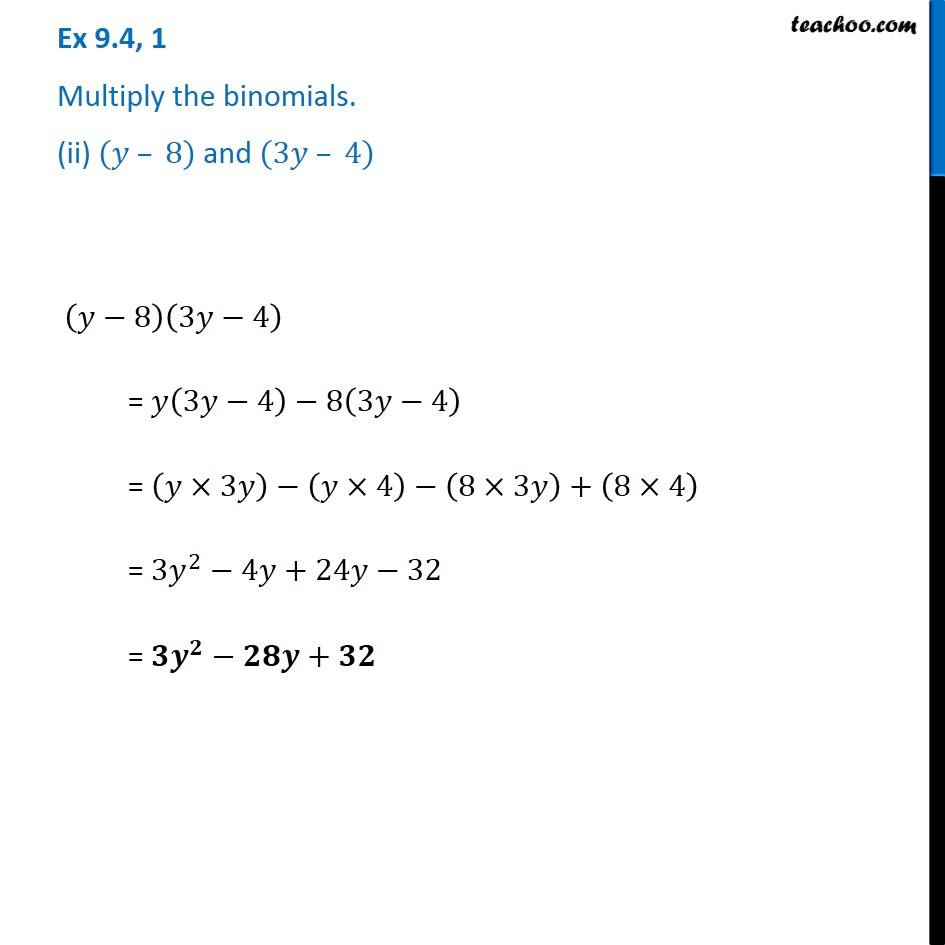Subscribe to our Youtube Channel - https://you.tube/teachoo

1. Chapter 9 Class 8 Algebraic Expressions and Identities
2. Serial order wise
3. Ex 9.4

Transcript

Ex 9.4, 1 Multiply the binomials. (ii) (𝑦 – 8) and (3𝑦 – 4) (𝑦−8)(3𝑦−4) = 𝑦(3𝑦−4)−8(3𝑦−4) = (𝑦×3𝑦)−(𝑦×4)−(8×3𝑦)+(8×4) = 3𝑦^2−4𝑦+24𝑦−32 = 𝟑𝒚^𝟐−𝟐𝟖𝒚+𝟑𝟐

Ex 9.4